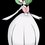# Solving quadratic or higher degree diophantine equations

I came across a problem in the set of number theory in which it involves solving of diophantine equations. (Especially this problem: https://brilliant.org/community-problem/a-number-theory-problem-by-souryajit-roy-2/?group=0RqFFfJZARDI&ref_id=211256) Some of the problems which involve diophantine equations of degree 2 or more are (really hard?) to solve. I tried to give information about the possible solutions and yes, I found some but I wasn't able to put all the solutions out. In despair, I used trial and error. And the worst case, I used Python to find all the solutions and it turned out there may be large increase from first solution to next solution. Do these kind of problems really require (not just for checking) programming language to get all the solutions? If not, what might be some techniques or theorems in which we are sure to get the solutions?Note by John Ashley Capellan
7 years, 1 month ago

This discussion board is a place to discuss our Daily Challenges and the math and science related to those challenges. Explanations are more than just a solution — they should explain the steps and thinking strategies that you used to obtain the solution. Comments should further the discussion of math and science.

When posting on Brilliant:

• Use the emojis to react to an explanation, whether you're congratulating a job well done , or just really confused .
• Ask specific questions about the challenge or the steps in somebody's explanation. Well-posed questions can add a lot to the discussion, but posting "I don't understand!" doesn't help anyone.
• Try to contribute something new to the discussion, whether it is an extension, generalization or other idea related to the challenge.
• Stay on topic — we're all here to learn more about math and science, not to hear about your favorite get-rich-quick scheme or current world events.

MarkdownAppears as
*italics* or _italics_ italics
**bold** or __bold__ bold
- bulleted- list
• bulleted
• list
1. numbered2. list
1. numbered
2. list
Note: you must add a full line of space before and after lists for them to show up correctly
paragraph 1paragraph 2

paragraph 1

paragraph 2

[example link](https://brilliant.org)example link
> This is a quote
This is a quote
    # I indented these lines
# 4 spaces, and now they show
# up as a code block.

print "hello world"
# I indented these lines
# 4 spaces, and now they show
# up as a code block.

print "hello world"
MathAppears as
Remember to wrap math in $$ ... $$ or $ ... $ to ensure proper formatting.
2 \times 3 $2 \times 3$
2^{34} $2^{34}$
a_{i-1} $a_{i-1}$
\frac{2}{3} $\frac{2}{3}$
\sqrt{2} $\sqrt{2}$
\sum_{i=1}^3 $\sum_{i=1}^3$
\sin \theta $\sin \theta$
\boxed{123} $\boxed{123}$

## Comments

Sort by:

Top Newest

If you look into the solution, I mentioned that we get a Pell's equation, whose solutions grow at an exponential rate. There is no need to do a computer search, if you understand the theory of Pell's equation.

Staff - 7 years, 1 month ago

Log in to reply

Is PE suitable for all higher degree ( 2+) diophantine equations, sir?

- 6 years, 11 months ago

Log in to reply

×

Problem Loading...

Note Loading...

Set Loading...